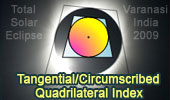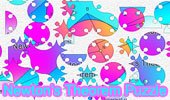# Online Geometry: Tangential or Circumscribed Quadrilateral, Theorems and ProblemsTangential or Circumscribed Quadrilateral, Theorems and Problems - IndexDynamic Geometry 1481. Five Tangential or Circumscribed Quadrilaterals, Pitot Theorem, Congruence, Step-by-step Illustration. GeoGebra, iPad. Dynamic Geometry 1470. Tangential Quadrilateral, Incircles, Tangent, Parallel, Rhombus, Step-by-step Illustration. Geometry Problem 1466. Tangential Quadrilateral, Newton Line, Incenter, Midpoint, Diagonal, Collinear Points. Step-by-step Illustration using GeoGebra. Geometry Problem 1465. Tangential Quadrilateral, Incenter, Inscribed Circle, Equal Sum of Areas. Step-by-step Illustration using GeoGebra. Geometry Problem 1351.Two Tangential or Circumscribed Quadrilaterals, Midpoints, Perpendiculars. Geometry Problem 1181 Cyclic Quadrilateral and Tangential Quadrilateral, Diameter as a Diagonal, Incenter, Circumcenter. Geometry Problem 910. Bicentric Quadrilateral, Distance between the Incenter and Circumcenter, Incircle, Circumcircle, Circumscribed, Inscribed, Circumradius. Geometry Problem 909. Bicentric Quadrilateral, Incircle, Circumcircle, Circumscribed, Inscribed, Tangent, Inradius, Distance. Geometry Problem 908. Bicentric Quadrilateral, Incircle, Circumcircle, Circumscribed, Inscribed, Tangent, Inradius. Geometry Problem 907. Bicentric Quadrilateral, Incircle, Circumcircle, Circumscribed, Inscribed, Tangent, Inradius. Geometry Problem 906. Bicentric Quadrilateral, Incircle, Circumcircle, Circumscribed, Inscribed, Perpendicular. Geometry Problem 883 Five Circumscribed Quadrilaterals, Circle, Tangent, Common Tangent. Geometry Problem 817.Two circles, Tangent Lines, Circumscribed Quadrilaterals. Geometry Problem 816. Tangential or Circumscribed Quadrilateral: Pitot Theorem Proposed Problem 371. Square, Inscribed circle, Triangle, Area. Proposed Problem 352. Tangential quadrilateral, Incircles, Common tangent, Circumscribable or Tangential quadrilateral. Proposed Problem 351. Rhombus, Incircles, Common tangent, Circumscribable or Tangential quadrilateral. Eight-Point Circle Theorem Step-by-Step construction, Manipulation, and animation. Geometry Problem 724. Circumscribed Quadrilateral, Circle, Angle, Incenter, Half the Measure. Proposed Problem 331. Square, Point on the Inscribed Circle, Tangency Points. Proposed Problem 322. Square, Inscribed circle, Tangent, Triangle area. Newton's Theorem: Newton's Line. Circumscribed quadrilateral, midpoints of diagonals, center of the circle inscribed. Proposition 7 Square and inscribed and circumscribed Circles Proposed Problem 153. Circumscribed Quadrilateral, Diagonals Concurrent with Chords. Proposed Problem 152. Circumscribed Quadrilateral, Diagonal, Chord, Proportion. Proposed Problem 76: Area of a Circle. Square, Circle, Circular Sector. Proposed Problem 62: Square Diagonal, Inscribed Circle.Puzzle of the Newton's Theorem: 50 pieces of circles.

 Home | Search | Geometry | Post a comment | Email | by Antonio GutierrezLast updated: May 24, 2020